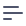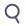# Getting Started with Cube.js

Cube.js is a framework used by data engineers to model raw data into meaningful business definitions and pre-aggregate and optimize results. By building data schema, you will provide your business users with self-service access to data and will create a single source of truth. By pre-aggregating and optimizing your schema, you will make this self-service access to data extremely fast.

Let’s use a users table with the following columns as an example:

idpayingcitycompany_name
1trueSan FranciscoPied Piper
2truePalo AltoRaviga
3trueRedwoodAvito
4falseMountain ViewBream-Hall
5falseSanta CruzHooli

We can start with a set of simple questions about users we want to answer:

• How many users do we have?
• How many paying users?
• What is the percentage of paying users out of the total?
• How many users, paying or not, are from different cities and companies?

We don’t need to write a SQL code for every question.
Cube.js is all about building well-organized and reusable SQL.

## 1. Creating a Cube

In Cube.js, cubes are used to organize entities and connections between entities. Usually one cube is created for each table in the database, such as `users`, `orders`, `products`, etc. In `sql` parameter of the cube we define a base table for this cube. In our case the base table is simply our `users` table.

``````cube(`Users`, {
sql: `SELECT * FROM users`
});
``````

## 2. Adding Measures and Dimensions

Once the base table is defined, the next step is to add measures and dimensions to the cube.

Measure is referred to as quantitative data, such as number of units sold, number of unique visits, profit, and so on.

Dimension is referred to as categorical data, such as state, gender, product name, or units of time (e.g., day, week, month).

Let's go ahead and create our first measure and two dimensions.

``````cube(`Users`, {
sql: `SELECT * FROM users`,

measures: {
count: {
sql: `id`,
type: `count`
},
},

dimensions: {
city: {
sql: `city`,
type: `string`
},

companyName: {
sql: `company_name`,
type: `string`
}
}
});
``````

Let's break down this code snippet by pieces. After defining the base table for the cube, we create a `count` measure in the Measures block. Type `count` and sql `id` means that when this measure is selected in the user interface, Cube.js will generate the following SQL:

``````SELECT count(id) from users;
``````

When we apply a city dimension to the measure to see "Where are users based?" Statsbot will generate SQL with a GROUP BY clause:

``````SELECT city, count(id) from users GROUP BY 1;
``````

You can add as many dimensions as you want when you perform grouping in the Statsbot interface.

## 3. Adding Filters to Measures

Now let's answer the next question – "How many paying users do we have?" To accomplish this we will introduce measure filters:

``````cube(`Users`, {
measures: {
count: {
sql: `id`,
type: `count`
},

payingCount: {
sql: `id`,
type: `count`,
filters: [
{ sql: `\${CUBE}.paying = 'true'` }
]
}
}
});
``````

It is best practice to prefix rerenfeces to table columns with the name of the cube or with CUBE constant when referencing the current cube's column.

That's it! Now we have the `payingCount` measure, which shows only our paying users. When this measure is selected in the user interface Statsbot will generate the following SQL:

``````SELECT
count(
CASE WHEN (users.paying = 'true') THEN users.id END
) "users.paying_count"
FROM users
``````

Since the filters property is an array, you can apply as many filters as you like. `payingCount` can be used with dimensions the same way as simple `count`. We can group `payingCount` by `city` and `companyName` simply by adding these dimensions alongside measures in the Statsbot interface.

## 4. Using Calculated Measures

To answer "What is the percentage of paying users out of the total?" we need to calculate the paying users ratio, which is basically `payingCount/count`. Cube.js makes it extremely easy to perform this kind of calculation. Let's add a new measure to our cube called `payingPercentage'

``````cube(`Users`, {
measures: {
count: {
sql: `id`,
type: `count`
},

payingCount: {
sql: `id`,
type: `count`,
filters: [
{ sql: `\${TABLE}.paying = 'true'` }
]
},

payingPercentage: {
sql: `100.0 * \${payingCount} / \${count}`,
type: `number`,
format: `percent`
}
}
});
``````

Here we defined a calculated measure, `payingPercentage`, which is basically a division of `payingCount` by `count`. This example shows how you can reference measures inside other measure definitions. When you select the `payingPercentage` measure inside the Statsbot interface, the following SQL will be generated:

``````SELECT
100.0 * count(
CASE WHEN (users.paying = 'true') THEN users.id END
) / count(users.id) "users.paying_percentage"
FROM users
``````

Same as for other measures, `payingPercentage` can be used with dimensions.

## 5. Next Steps

We've answered all our questions in the beginning of the tutorial. But there is a lot more Cube.js can do for you. We recommend checking out the Reference documentation, as well as Guides and Tutorials.

And please don't hesitate to contact the Statsbot team. Click the circle in the bottom right corner of the page, anywhere on the site, to get support and help with your questions.